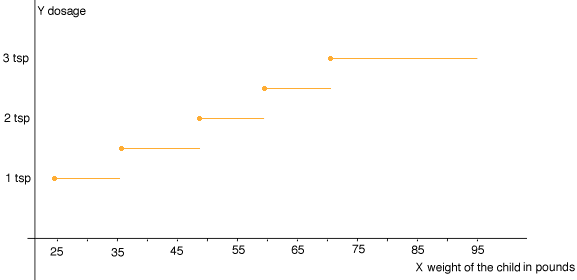Quandaries and Queries My name is Duncan. This is an extra credit question in our advanced algebra class. The dosage on the box I found read: 24-35 lbs (1 tsp),36-47 lbs(1.5tsp),48-59 (2tsp),60-71(2.5),72-95(3tsp). thanks.....Duncan Introduction                We deal with piecewise functions when we look at over the counter drugs – cough medicine, Tylenol – particularly for children.     Scenario                      A child is assigned to your care and she has a headache.  The parent has authorized the administration of Children’s Tylenol.  Read a box of Children’s Tylenol (or any other similar drug) and find the directions for administration.  Note the child’s weight and the corresponding dosage. (Be sure the drug you choose has a minimum of 4 weight intervals.)   Directions                   Part A 1.   Create a table for the data where X = weight interval of child Y = corresponding drug dosage 2.      Graph the information on graph paper. You should have a piecewise function. 3.      Name the Domain for the function. 4.      Name the Range for the function.     Part B   1.      Create a table for the same data, however let X= minimum weight in the weight interval Y= corresponding drug dosage. 2.   Create a scatter plot for the data. 3.      Name the Domain. 4.      Name the Range. 5.      Create a line graph for the data. 6.      Find a linear equation for the line. (Hint:  Use the form  y - y1 = m(x - x1) to find the equation.) 7.      Use the model from question 6 to determine symbolically, the number of tablets that should be administered to a child weighing 35 pounds. Hi Duncan, I'll help you get started. When I set out to plot the graph asked for in part A2 I wondered what to do with a child who weight 35 and a half pounds. I decided that to be safe I wouldn't give a 1.5 tsp to my child unless he were 36 pounds so I plotted the first line (1 tsp) from 24 pounds to as close to 36 pounds as I could get and the at 36 pounds I started the next line segment at 1.5 tsp. So here is my graph.I put a dot at the left end of each line segment since that gives the scatter diagram asked for in part B. Can you see now how to proceed? Penny Duncan wrote back Can you help me with 6 and 7 in Part B. please...Duncan I would draw the graph called for in part B2 on graph paper, as accurately as possible. Then take a ruler and see if the points lie on a straight line. They probably don't but they will be very close. Use your judgement and choose two of the points where the line through these points comes closest to going through all of the points. Using these two points write the equation of the line. Substitute x = 35 pounds into this equation to see what value you get for y. Penny